Discussion

# Bill Generation using Integer Optimisation

Linear Programming is a powerful method for finding optimised solutions. It is intricately complex and needs specialised study. However, simple and everyday use tools like Microsoft Excel can be used to solve reasonably complex Integer Optimisation problems.

Below is an illustration. For purpose of illustration, the problem has been simplified and the number of variables and constraints has been kept small.

We consider a Telecom Company which needs issuing Bills to its 30,00,000 (30 Lakhs or 3 million) Customers. Suppose that the Telecom Company uses 4 machines for generating the bills. Each machine has different capacities for Bill Generation as is given in the table below.Further suppose that the Telecom Company has divided its Customers into 10 Bill Cities or Bill Zones. This implies that the Telecom Company would like to generate the Bills for all the Customers of a single Bill City through a single process. So, all the Bills for a Bill City should be processed by a single machine. The number of Customers in the different Bill Cities is given below.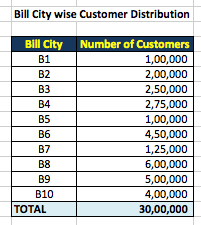Our problem is to assign each Bill City to a Machine such that the total number of hours required for the entire Bill Generation is the least.

We consider the variable X(i,j) where i is the index for Bill City and j is the index for Machine. So, X(1,1) denotes the variable for Bill City B1 and Machine A. Now, X(1,1) will take a value of 1 if the Bill City B1 is assigned to Machine A, else X(1,1) will take a value of 0. Similarly, all the variables X(i,j) will take a value of 1 if the i th Bill City is assigned to the j th Machine, else X(i,j) will take a value of 0. i can vary from 1 to 10 and j can vary from 1 to 4 in our example.

The picture below displays our decision variables. Each of the cells in yellow can take a value of 0 or 1.This seems like a simple problem and should be solvable by hand. However, it turns out that to find the optimum solution for this simple problem can take a lot of time if solved by hand.

To solve using Linear Programming, we need first defining our Objective Function. The Objective Function can be either maximised or minimised. As stated earlier, our objective is to minimise the number of hours required to generate all the bills. For this, we first compute the number of hours required for the generation of the bills for each Bill City on each Machine.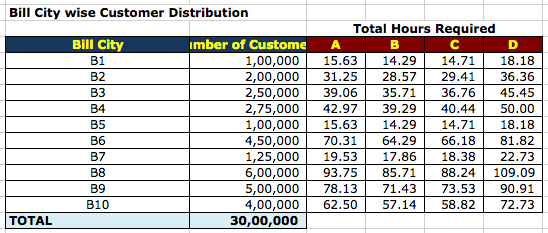Let us call these values under the “Total Hours Required” columns as H(i,j). So, H(1,3) is the number of hours required for processing all the Bills of Bill City B1 using Machine C.

Now, if Bill City is assigned to Machine A, then the number of hours required would be X(1,1) * H(1,1). Similarly, if Bill City B9 is assigned to Machine B, then the number of hours required would be X(9,2) * H(9,2).

But X(i,j) can take a value of 0 or 1. So, either X(1,1) * H(1,1) can be 0 hours or 15.63 hours. In other words, the number of hours would be counted (or rather required) only if the Bill City is assigned to a particular Machine.

So, our objective function is the summation of all X(i,j) * H(i,j) over all i and j. This can be computed in Microsoft Excel using the SUMPRODUCT function.

Lastly, we need defining the constraints to our model. The initial constraints are as follows.

• We know that each Bill City should be processed on exactly one machine. So, for Bill City B1, either X(1,1) is 1 or X(1,2) is 1 or X(1,3) or X(1,4) is 1. This can be defines as a constraint as X(1,1) + X(1,2) + X(1,3) + X(1,4) = 1.  Similarly, for Bill City B2, the constraint is X(2,1) + X(2,2) + X(2,3) + X(2,4) = 1. Similarly, for Bill City B3, the constraint is X(3,1) + X(3,2) + X(3,3) + X(3,4) = 1. And so on for all the Bill Cities. So, we have a total of 10 constraints of this nature.
• We add a further constraint that all the Bills should be generated in 5 days or 120 hours. So, the maximum running time of any machine has to be less than or equal (<=) to 120. For Machine A, this can be formulated as X(1,1) * H(1,1) + X(2,1) * H(2,1) + X(3,1) * H(3,1) + X(4,1) * H(4,1) + X(5,1) * H(5,1) + X(6,1) * (H(6,1) + X(7,1) * H(7,1) + X(8,1) * H(8,1) + X(9,1) * H(9,1) + X(10,1) * H(10,1) <= 120. Ans so on for all the Machines. So, we will have a total of 4 constraints of this nature.
• One additional constraint in this case needs adding to indicate that all the decision variables are binary.

Linear Programming using Microsoft Excel can be done using the Solver feature. Solver can be found under Tools (so, Tools -> Solver). If Solver is not found under Tools, it can be added using Tools -> Add Ins…

In the Solver, define the Objective Function, the Decision Variables and the Constraints. Select optimisation need as Minimise in this case. Then, select “Simplex LP” as the Solving Method. And click Solve. The solution obtained is displayed below.So, each Bill City is assigned to exactly one Machine. The total capacity processed by each Machine is provided below.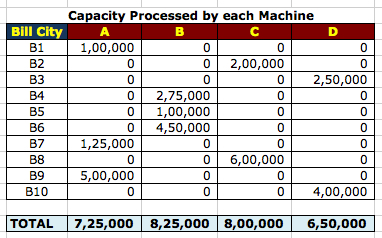The total time each Machine will be run is given below.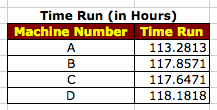Now, suppose we add a constraint that Machine A should not process more than 2 Bill Cities. This can be added as a constraint as follows. X(1,1) + X(2,1) + X(3,1) + X(4,1) + X(5,1) + X(6,1) + X(7,1) + X(8,1) + X(9,1) + X(10,1) <= 2.

On adding this constraint and solving, we get the following assignment. The assignments have changed from before and the constraint has been accommodated.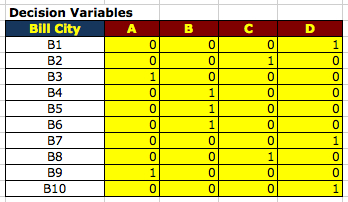The Capacity Processed by each Machine and the Run Time of each Machine is as follows.Categories: Discussion, Technical

1.SutoCom says: